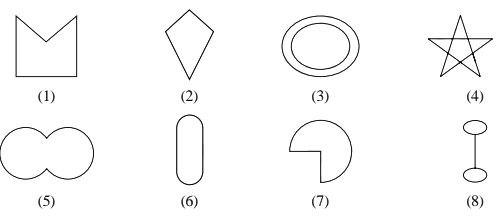Following are some figures: Classify each of these figures on the basis of the following:Polygon

# Following are some figures: Classify each of these figures on the basis of the following:Polygon1. A
Figures (1), (2), (5), (6), and (7).
2. B
Figures (1), and (2)
3. C
Figures (1), (2), (5), (6), and (8).
4. D
Figures (3), (4), (5), (6), and (7)

Fill Out the Form for Expert Academic Guidance!l

+91

Live ClassesBooksTest SeriesSelf Learning

Verify OTP Code (required)

### Solution:

Given,
We have to find polygon.
In geometry, polygon is defined as a two-dimensional shape having three or more sides that are closed by straight lines only.
Therefore, figures (1) and (2) are polygons.
Hence, the correct option is 2.

## Related content

 Area of Square Area of Isosceles Triangle Pythagoras Theorem Triangle Formula Perimeter of Triangle Formula Area Formulae Volume of Cone Formula Matrices and Determinants_mathematics Critical Points Solved Examples Type of relations_mathematics+91

Live ClassesBooksTest SeriesSelf Learning

Verify OTP Code (required)